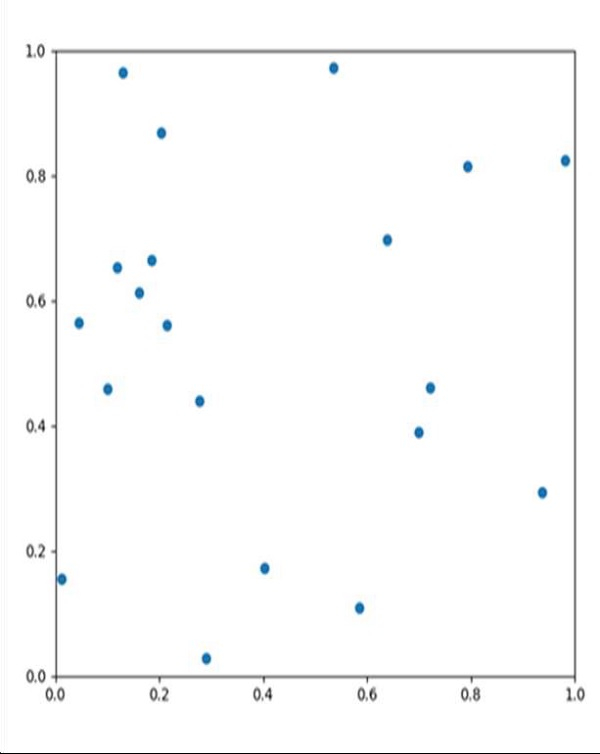# How to animate a scatter plot in Matplotlib?

Using the FuncAnimation method of matplotlib, we can animate the diagram. We can pass a user defined method where we will be changing the position of the particles, and at the end, we will return plot type.

## Steps

• Get the particle's initial position, velocity, force, and size.

• Create a new figure, or activate an existing figure with figsize = (7, 7).

• Add an axes to the current figure and make it the current axes, with xlim and ylim.

• Plot scatter for initial position of the particles.

• Make an animation by repeatedly calling a function *func*. We can pass a user-defined method that helps to change the position of particles, into the FuncAnimation class.

• Using plt.show(), show the figure.

## Example

import matplotlib.pyplot as plt
from matplotlib.animation import FuncAnimation
import numpy as np

dt = 0.005
n=20
L = 1
particles=np.zeros(n,dtype=[("position", float , 2),
("velocity", float ,2),
("force", float ,2),
("size", float , 1)])

particles["position"]=np.random.uniform(0,L,(n,2));
particles["velocity"]=np.zeros((n,2));
particles["size"]=0.5*np.ones(n);

fig = plt.figure(figsize=(7,7))
ax = plt.axes(xlim=(0,L),ylim=(0,L))
scatter=ax.scatter(particles["position"][:,0], particles["position"][:,1])

def update(frame_number):
particles["force"]=np.random.uniform(-2,2.,(n,2));
particles["velocity"] = particles["velocity"] + particles["force"]*dt
particles["position"] = particles["position"] + particles["velocity"]*dt

particles["position"] = particles["position"]%L
scatter.set_offsets(particles["position"])
return scatter,

anim = FuncAnimation(fig, update, interval=10)
plt.show()

## Output